## DBSCAN

### Introduction to DBSCAN

Density-Based Spatial Clustering of Applications with Noise (DBSCAN) identifies arbitrarily shaped clusters and noise (outliers) in data. The Statistics and Machine Learning Toolbox™ function `dbscan` performs clustering on an input data matrix or on pairwise distances between observations. `dbscan` returns the cluster indices and a vector indicating the observations that are core points (points inside clusters). Unlike k-means clustering, the DBSCAN algorithm does not require prior knowledge of the number of clusters, and clusters are not necessarily spheroidal. DBSCAN is also useful for density-based outlier detection, because it identifies points that do not belong to any cluster.

For a point to be assigned to a cluster, it must satisfy the condition that its epsilon neighborhood (`epsilon`) contains at least a minimum number of neighbors (`minpts`). Or, the point can lie within the epsilon neighborhood of another point that satisfies the `epsilon` and `minpts` conditions. The DBSCAN algorithm identifies three kinds of points:

• Core point — A point in a cluster that has at least `minpts` neighbors in its epsilon neighborhood

• Border point — A point in a cluster that has fewer than `minpts` neighbors in its epsilon neighborhood

• Noise point — An outlier that does not belong to any cluster

DBSCAN works with a wide range of distance metrics, and you can define a custom distance metric for your particular application. The choice of a distance metric determines the shape of the neighborhood.

### Algorithm Description

For specified values of the epsilon neighborhood `epsilon` and the minimum number of neighbors `minpts` required for a core point, the `dbscan` function implements DBSCAN as follows:

1. From the input data set `X`, select the first unlabeled observation x1 as the current point, and initialize the first cluster label C to 1.

2. Find the set of points within the epsilon neighborhood `epsilon` of the current point. These points are the neighbors.

1. If the number of neighbors is less than `minpts`, then label the current point as a noise point (or an outlier). Go to step 4.

Note

`dbscan` can reassign noise points to clusters if the noise points later satisfy the constraints set by `epsilon` and `minpts` from some other point in `X`. This process of reassigning points happens for border points of a cluster.

2. Otherwise, label the current point as a core point belonging to cluster C.

3. Iterate over each neighbor (new current point) and repeat step 2 until no new neighbors are found that can be labeled as belonging to the current cluster C.

4. Select the next unlabeled point in `X` as the current point, and increase the cluster count by 1.

5. Repeat steps 2–4 until all points in `X` are labeled.

### Determine Values for DBSCAN Parameters

This example shows how to select values for the `epsilon` and `minpts` parameters of `dbscan`. The data set is a Lidar scan, stored as a collection of 3-D points, that contains the coordinates of objects surrounding a vehicle.

Load, preprocess, and visualize the data set.

Load the x, y, z coordinates of the objects.

```load('lidar_subset.mat') X = lidar_subset;```

To highlight the environment around the vehicle, set the region of interest to span 20 meters to the left and right of the vehicle, 20 meters in front and back of the vehicle, and the area above the surface of the road.

```xBound = 20; % in meters yBound = 20; % in meters zLowerBound = 0; % in meters```

Crop the data to contain only points within the specified region.

```indices = X(:,1) <= xBound & X(:,1) >= -xBound ... & X(:,2) <= yBound & X(:,2) >= -yBound ... & X(:,3) > zLowerBound; X = X(indices,:); ```

Visualize the data as a 2-D scatter plot. Annotate the plot to highlight the vehicle.

```scatter(X(:,1),X(:,2),'.'); annotation('ellipse',[0.48 0.48 .1 .1],'Color','red')```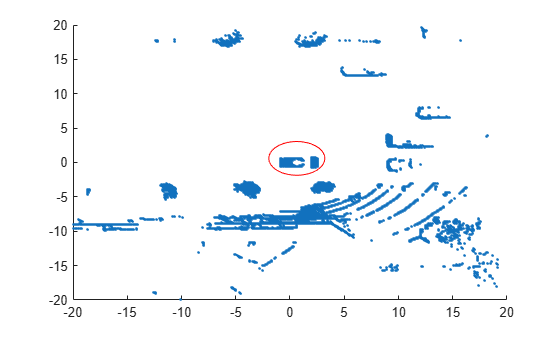The center of the set of points (circled in red) contains the roof and hood of the vehicle. All other points are obstacles.

Select a value for `minpts`.

To select a value for `minpts`, consider a value greater than or equal to one plus the number of dimensions of the input data . For example, for an `n`-by-`p` matrix `X`, set the value of `'minpts'` greater than or equal to p`+1`.

For the given data set, specify a `minpts` value greater than or equal to 4, specifically the value 50.

`minpts = 50; % Minimum number of neighbors for a core point`

Select a value for `epsilon`.

One strategy for estimating a value for `epsilon` is to generate a k-distance graph for the input data `X`. For each point in `X`, find the distance to the kth nearest point, and plot sorted points against this distance. The graph contains a knee. The distance that corresponds to the knee is generally a good choice for `epsilon`, because it is the region where points start tailing off into outlier (noise) territory .

Before plotting the k-distance graph, first find the `minpts` smallest pairwise distances for observations in `X`, in ascending order.

`kD = pdist2(X,X,'euc','Smallest',minpts);`

Plot the k-distance graph.

```plot(sort(kD(end,:))); title('k-distance graph') xlabel('Points sorted with 50th nearest distances') ylabel('50th nearest distances') grid```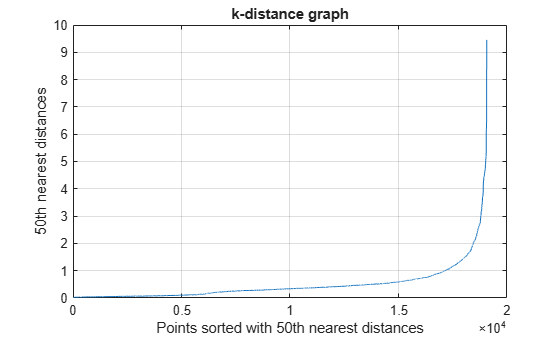The knee appears to be around 2; therefore, set the value of `epsilon` to 2.

`epsilon = 2;`

Cluster using `dbscan`.

Use `dbscan` with the values of `minpts` and `epsilon` that were determined in the previous steps.

`labels = dbscan(X,epsilon,minpts);`

Visualize the clustering and annotate the figure to highlight specific clusters.

```numGroups = length(unique(labels)); gscatter(X(:,1),X(:,2),labels,hsv(numGroups)); title('epsilon = 2 and minpts = 50') grid annotation('ellipse',[0.54 0.41 .07 .07],'Color','red') annotation('ellipse',[0.53 0.85 .07 .07],'Color','blue') annotation('ellipse',[0.39 0.85 .07 .07],'Color','black')```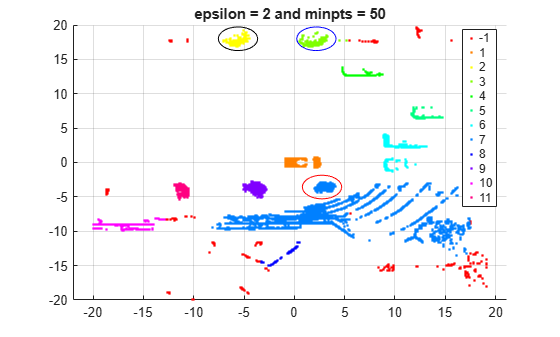`dbscan` identifies 11 clusters and a set of noise points. The algorithm also identifies the vehicle at the center of the set of points as a distinct cluster.

`dbscan` identifies some distinct clusters, such as the cluster circled in black (and centered around (–6,18)) and the cluster circled in blue (and centered around (2.5,18)). The function also assigns the group of points circled in red (and centered around (`3,–4`)) to the same cluster (group 7) as the group of points in the southeast quadrant of the plot. The expectation is that these groups should be in separate clusters.

Use a smaller value for `epsilon` to split up large clusters and further partition the points.

```epsilon2 = 1; labels2 = dbscan(X,epsilon2,minpts);```

Visualize the clustering and annotate the figure to highlight specific clusters.

```numGroups2 = length(unique(labels2)); gscatter(X(:,1),X(:,2),labels2,hsv(numGroups2)); title('epsilon = 1 and minpts = 50') grid annotation('ellipse',[0.54 0.41 .07 .07],'Color','red') annotation('ellipse',[0.53 0.85 .07 .07],'Color','blue') annotation('ellipse',[0.39 0.85 .07 .07],'Color','black')```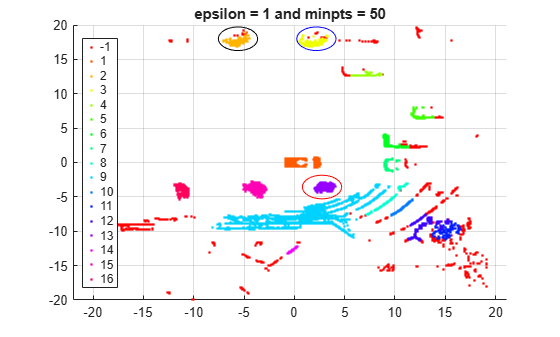By using a smaller epsilon value, `dbscan` is able to assign the group of points circled in red to a distinct cluster (group 13). However, some clusters that `dbscan` correctly identified before are now split between cluster points and outliers. For example, see cluster group 2 (circled in black) and cluster group 3 (circled in blue). The correct `epsilon` value is somewhere between 1 and 2.

Use an `epsilon` value of `1.55` to cluster the data.

```epsilon3 = 1.55; labels3 = dbscan(X,epsilon3,minpts);```

Visualize the clustering and annotate the figure to highlight specific clusters.

```numGroups3 = length(unique(labels3)); gscatter(X(:,1),X(:,2),labels3,hsv(numGroups3)); title('epsilon = 1.55 and minpts = 50') grid annotation('ellipse',[0.54 0.41 .07 .07],'Color','red') annotation('ellipse',[0.53 0.85 .07 .07],'Color','blue') annotation('ellipse',[0.39 0.85 .07 .07],'Color','black')```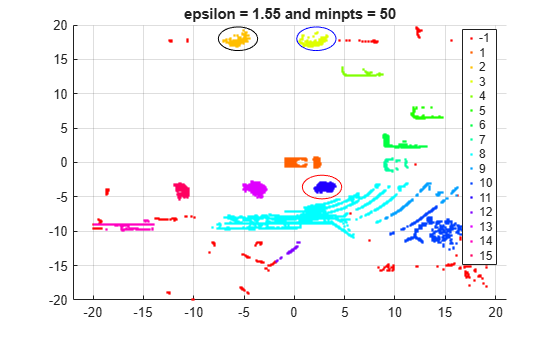`dbscan` does a better job of identifying the clusters when `epsilon` is set to `1.55`. For example, the function identifies the distinct clusters circled in red, black, and blue (with centers around (`3,–4`), (–6,18), and (2.5,18), respectively).

 Ester, M., H.-P. Kriegel, J. Sander, and X. Xiaowei. “A density-based algorithm for discovering clusters in large spatial databases with noise.” In Proceedings of the Second International Conference on Knowledge Discovery in Databases and Data Mining, 226-231. Portland, OR: AAAI Press, 1996.